Most Affordable JEE | NEET | 8,9,10 Preparation by Kota's Top IITian Doctor Faculties

# NCERT Solutions for Class 12 Physics Chapter 9 Ray Optics and Optical Instruments PDF - eSaral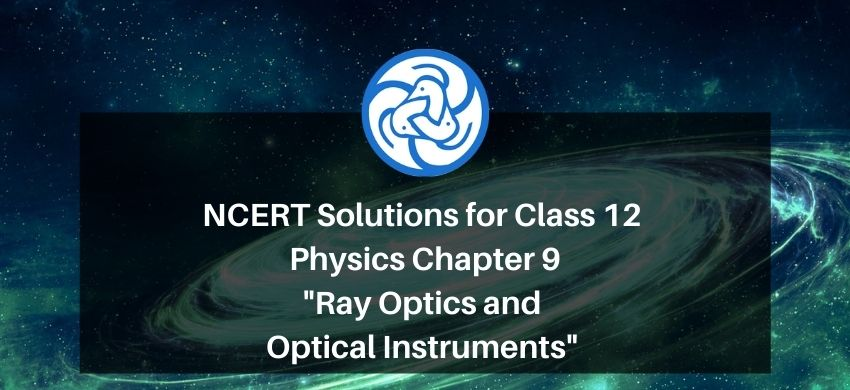`

# NCERT Solutions for Class 12 Physics Chapter 9 Ray Optics and Optical Instruments PDF

Hey, are you a class 12 student and looking for ways to download NCERT Solutions for Class 12 Physics Chapter 9 Ray Optics and Optical Instruments PDF? If yes. Then read this post till the end.

In this article, we have listed NCERT Solutions for Class 12 Physics Chapter 9 Ray Optics and Optical Instruments in PDF that are prepared by Kota’s top IITian Faculties by keeping Simplicity in mind.

If you want to learn and understand class 12 Physics Chapter 9 "Ray Optics and Optical Instruments" in an easy way then you can use these solutions PDF.

NCERT Solutions helps students to Practice important concepts of subjects easily. Class 12 Physics solutions provide detailed explanations of all the NCERT questions that students can use to clear their doubts instantly.

If you want to score high in your class 12 Physics Exam then it is very important for you to have a good knowledge of all the important topics, so to learn and practice those topics you can use eSaral NCERT Solutions.

In this article, we have listed NCERT Solutions for Class 12 Physics Chapter 9 Ray Optics and Optical Instruments PDF that you can download to start your preparations anytime.

So, without wasting more time Let’s start.

### Download NCERT Solutions for Class 12 Physics Chapter 9 Ray Optics and Optical Instruments PDF

Question 1: A small candle, $2.5 \mathrm{~cm}$ in size is placed at $27 \mathrm{~cm}$ in front of a concave mirror of radius of curvature $36 \mathrm{~cm}$. At what distance from the mirror should a screen be placed in order to obtain a sharp image? Describe the nature and size of the image. If the candle is moved closer to the mirror, how would the screen have to be moved?

Solution. Size of the candle, $h=2.5 \mathrm{~cm}$

Image size $=h^{\prime}$

Object distance, $u=-27 \mathrm{~cm}$

Radius of curvature of the concave mirror, $R=-36 \mathrm{~cm}$

Focal length of the concave mirror, $f=\frac{R}{2}=-18 \mathrm{~cm}$

Image distance $=v$

The image distance can be obtained using the mirror formula:

$\frac{1}{u}+\frac{1}{v}=\frac{1}{f}$

$\frac{1}{v}=\frac{1}{f}-\frac{1}{u}$

$=\frac{1}{-18}-\frac{1}{-27}=\frac{-3+2}{54}=-\frac{1}{54}$

$\therefore v=-54 \mathrm{~cm}$

Therefore, the screen should be placed $54 \mathrm{~cm}$ away from the mirror to obtain a sharp image.

The magnification of the image is given as:

$m=\frac{h^{\prime}}{h}=-\frac{v}{u}$

$\therefore h^{\prime}=-\frac{v}{u} \times h$

$=-\left(\frac{-54}{-27}\right) \times 2.5=-5 \mathrm{~cm}$

The height of the candle's image is $5 \mathrm{~cm}$. The negative sign indicates that the image is inverted and real.

If the candle is moved closer to the mirror, then the screen will have to be moved away from the mirror in order to obtain the image.

Question 2: A $4.5 \mathrm{~cm}$ needle is placed $12 \mathrm{~cm}$ away from a convex mirror of focal length $15 \mathrm{~cm}$. Give the location of the image and the magnification. Describe what happens as the needle is moved farther from the mirror.

Solution. Height of the needle, $h_{1}=4.5 \mathrm{~cm}$

Object distance, $u=-12 \mathrm{~cm}$

Focal length of the convex mirror, $f=15 \mathrm{~cm}$

Image distance $=v$

The value of $v$ can be obtained using the mirror formula:

$\frac{1}{u}+\frac{1}{v}=\frac{1}{f}$

$\frac{1}{v}=\frac{1}{f}-\frac{1}{u}$

$=\frac{1}{15}+\frac{1}{12}=\frac{4+5}{60}=\frac{9}{60}$

$\therefore v=\frac{60}{9}=6.7 \mathrm{~cm}$

Hence, the image of the needle is $6.7 \mathrm{~cm}$ away from the mirror. Also, it is on the other side of the mirror.

The image size is given by the magnification formula:

$m=\frac{h_{2}}{h_{1}}=-\frac{v}{u}$

$\therefore h_{2}=-\frac{v}{u} \times h_{1}$

$=\frac{-6.7}{-12} \times 4.5=+2.5 \mathrm{~cm}$

Hence, magnification of the image, $m=\frac{h_{2}}{h_{1}}=\frac{2.5}{4.5}=0.56$

The height of the image is $2.5 \mathrm{~cm}$. The positive sign indicates that the image is erect, virtual, and diminished.

If the needle is moved farther from the mirror, the image will also move away from the mirror, and the size of the image will reduce gradually.

Question 3: index of water? If water is replaced by a liquid of refractive index $1.63$ up to the same height, by what distance would the microscope have to be moved to focus on the needle again?

Solution. Actual depth of the needle in water, $h_{1}=12.5 \mathrm{~cm}$

Apparent depth of the needle in water, $h_{2}=9.4 \mathrm{~cm}$

Refractive index of water $=\mu$

The value of $\mu$ can be obtained as follows:

$\mu=\frac{h_{1}}{h_{2}}$

$=\frac{12.5}{9.4} \approx 1.33$

Hence, the refractive index of water is about $1.33$.

Water is replaced by a liquid of refractive index, $\mu^{\prime}=1.63$

The actual depth of the needle remains the same, but its apparent depth changes. Let $y$ be the new apparent depth of the needle. Hence, we can write the relation:

$\mu^{\prime}=\frac{h_{1}}{y}$

$\therefore y=\frac{h_{1}}{\mu^{\prime}}$

$=\frac{12.5}{1.63}=7.67 \mathrm{~cm}$

Hence, the new apparent depth of the needle is $7.67 \mathrm{~cm}$. It is less than $h_{2}$. Therefore, to focus the needle again, the microscope should be moved up

$\therefore$ Distance by which the microscope should be moved up $=9.4-7.67$

$=1.73 \mathrm{~cm}$

Question 4: Figures 9.34(a) and (b) show refraction of a ray in air incident at 60° with the normal to a glass-air and water-air interface, respectively. Predict the angle of refraction in glass when the angle of incidence in water is $45^{\circ}$ with the normal to a water-glass interface [Fig. $\left.9.34(\mathrm{c})\right]$.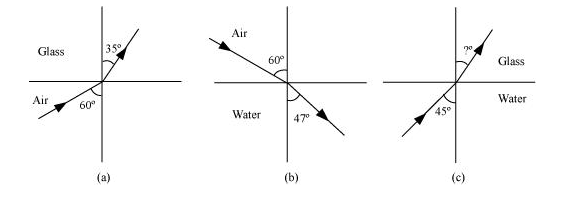Solution. As per the given figure, for the glass - air interface:

Angle of incidence, $i=60^{\circ}$

Angle of refraction, $r=35^{\circ}$

The relative refractive index of glass with respect to air is given by Snell's law as:

$\mu_{\mathrm{g}}^{\mathrm{a}}=\frac{\sin i}{\sin \gamma}$

$=\frac{\sin 60^{\circ}}{\sin 35^{\circ}}=\frac{0.8660}{0.5736}=1.51$$\ldots(1) As per the given figure, for the air - water interface: Angle of incidence, i=60^{\circ} The relative refractive index of water with respect to air is given by Snell's law as: \mu_{w}^{\mathrm{a}}=\frac{\sin i}{\sin r} =\frac{\sin 60}{\sin 47}=\frac{0.8660}{0.7314}=1.184$$\ldots(2)$

Using (1) and (2), the relative refractive index of glass with respect to water can be obtained as:

$\mu_{\mathrm{g}}^{\mathrm{w}}=\frac{\mu_{\mathrm{g}}^{\mathrm{a}}}{\mu_{\mathrm{w}}^{\mathrm{a}}}$

$=\frac{1.51}{1.184}=1.275$

The following figure shows the situation involving the glass − water interface.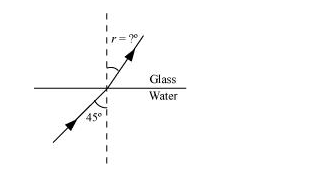Angle of incidence, $i=45^{\circ}$

Angle of refraction $=r$

From Snell's law, $r$ can be calculated as:

$\frac{\sin i}{\sin r}=\mu_{\mathrm{g}}^{\mathrm{w}}$

$\frac{\sin 45^{\circ}}{\sin r}=1.275$’

$\sin r=\frac{\frac{1}{\sqrt{2}}}{1.275}=0.5546$

$\therefore r=\sin ^{-1}(0.5546)=38.68^{\circ}$

Hence the anale of refraction at the water - alass interface is $3868^{\circ}$

Question 5: A small bulb is placed at the bottom of a tank containing water to a depth of $80 \mathrm{~cm}$. What is the area of the surface of water through which light from the bulb can emerge out? Refractive index of water is $1.33$. (Consider the bulb to be a point source.)

Solution. Actual depth of the bulb in water, $d_{1}=80 \mathrm{~cm}=0.8 \mathrm{~m}$

Refractive index of water, $\mu=1.33$

The given situation is shown in the following figure: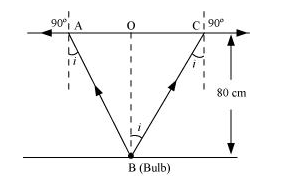Where,

$i=$ Angle of incidence

$r=$ Angle of refraction $=90^{\circ}$

Since the bulb is a point source, the emergent light can be considered as a circle of radius, $R=\frac{A C}{2}=A O=O B$

Using Snell’ law, we can write the relation for the refractive index of water as:

$\mu=\frac{\sin r}{\sin i}$

$1.33=\frac{\sin 90^{\circ}}{\sin i}$

$\therefore i=\sin ^{-1}\left(\frac{1}{1.33}\right)=48.75^{\circ}$

Using the given figure, we have the relation:

$\tan i=\frac{O C}{O B}=\frac{R}{d_{1}}$

$\therefore R=\tan 48.75^{\circ} \times 0.8=0.91 \mathrm{~m}$

$\therefore$ Area of the surface of water $=\pi R^{2}=\pi(0.91)^{2}=2.61 \mathrm{~m}^{2}$

Hence, the area of the surface of water through which the light from the bulb can emerge is approximately $2.61 \mathrm{~m}^{2}$.

Question 6: A prism is made of glass of unknown refractive index. A parallel beam of light is incident on a face of the prism. The angle of minimum deviation is measured to be $40^{\circ}$. What is the refractive index of the material of the prism? The refracting angle of the prism is $60^{\circ}$. If the prism is placed in water (refractive index 1.33), predict the new angle of minimum deviation of a parallel beam of light.

Solution. Angle of minimum deviation, $\delta_{\mathrm{m}}=40^{\circ}$

Angle of the prism, $A=60^{\circ}$

Refractive index of water, $\mu=1.33$ Refractive index of the material of the prism $=\mu^{\prime}$

The angle of deviation is related to refractive index $\left(\mu^{\prime}\right)$ as:

$\mu^{\prime}=\frac{\sin \frac{\left(A+\delta_{m}\right)}{2}}{\sin \frac{A}{2}}$

$=\frac{\sin \frac{\left(60^{\circ}+40^{\circ}\right)}{2}}{\sin \frac{60^{\circ}}{2}}=\frac{\sin 50^{\circ}}{\sin 30^{\circ}}=1.532$

Hence, the refractive index of the material of the prism is $1.532$.

Since the prism is placed in water, let $\delta_{\mathrm{m}}{ }^{\prime}$ be the new angle of minimum deviation for the same prism.

The refractive index of glass with respect to water is given by the relation:

$\mu_{\mathrm{g}}^{\mathrm{W}}=\frac{\mu^{\prime}}{\mu}=\frac{\sin \frac{\left(A+\delta_{\mathrm{m}}^{\prime}\right)}{2}}{\sin \frac{A}{2}}$

$\sin \frac{\left(A+\delta_{\mathrm{m}}^{\prime}\right)}{2}=\frac{\mu^{\prime}}{\mu} \sin \frac{A}{2}$

$\sin \frac{\left(A+\delta_{\mathrm{m}}^{\prime}\right)}{2}=\frac{1.532}{1.33} \times \sin \frac{60^{\circ}}{2}=0.5759$

$\frac{\left(A+\delta_{\mathrm{m}}^{\prime}\right)}{2}=\sin ^{-1} 0.5759=35.16^{\circ}$

$60^{\circ}+\delta_{\mathrm{m}}^{\prime}=70.32^{\circ}$

$\therefore \delta_{\mathrm{m}}^{\prime}=70.32^{\circ}-60^{\circ}=10.32^{\circ}$

Hence, the new minimum angle of deviation is $10.32^{\circ}$.

Question 7: Double-convex lenses are to be manufactured from a glass of refractive index 1.55, with both faces of the same radius of curvature. What is the radius of curvature required if the focal length is to be $20 \mathrm{~cm} ?$

Solution. Refractive index of alass. $u=1.55$

Focal length of the double-convex lens, $f=20 \mathrm{~cm}$

Radius of curvature of one face of the lens $=R_{1}$

Radius of curvature of the other face of the lens $=R_{2}$

Radius of curvature of the double-convex lens $=R$

$\therefore R_{1}=R$ and $R_{2}=-R$

The value of $R$ can be calculated as:

$\frac{1}{f}=(\mu-1)\left[\frac{1}{R_{1}}-\frac{1}{R_{2}}\right]$

$\frac{1}{20}=(1.55-1)\left[\frac{1}{R}+\frac{1}{R}\right]$

$\frac{1}{20}=0.55 \times \frac{2}{R}$

$\therefore R=0.55 \times 2 \times 20=22 \mathrm{~cm}$

The value of R can be calculated as:

$\frac{1}{f}=(\mu-1)\left[\frac{1}{R_{1}}-\frac{1}{R_{2}}\right]$

$\frac{1}{20}=(1.55-1)\left[\frac{1}{R}+\frac{1}{R}\right]$

$\therefore R=0.55 \times 2 \times 20=22 \mathrm{~cm}$

Hence, the radius of curvature of the double-convex lens is $22 \mathrm{~cm}$.

Question 8: A beam of light converges at a point $P$. Now a lens is placed in the path of the convergent beam $12 \mathrm{~cm}$ from $P$. At what point does the beam converge if the lens is (a) a convex lens of focal length $20 \mathrm{~cm}$, and (b) a concave lens of focal length $16 \mathrm{~cm} ?$

Solution. In the given situation, the object is virtual and the image formed is real.

Object distance, $u=+12 \mathrm{~cm}$

(a) Focal length of the convex lens, $f=20 \mathrm{~cm}$

Image distance $=v$

According to the lens formula, we have the relation:

$\frac{1}{v}-\frac{1}{u}=\frac{1}{f}$

$\frac{1}{v}-\frac{1}{12}=\frac{1}{20}$

$\frac{1}{v}=\frac{1}{20}+\frac{1}{12}=\frac{3+5}{60}=\frac{8}{60}$

$\therefore v=\frac{60}{8}=7.5 \mathrm{~cm}$

Hence, the image is formed 7.5 cm away from the lens, toward its right.

(b) Focal length of the concave lens, $f=-16 \mathrm{~cm}$

Image distance $=v$

According to the lens formula, we have the relation:

$\frac{1}{v}-\frac{1}{u}=\frac{1}{f}$

$\frac{1}{v}=-\frac{1}{16}+\frac{1}{12}=\frac{-3+4}{48}=\frac{1}{48}$

$\therefore v=48 \mathrm{~cm}$

Hence, the image is formed 48 cm away from the lens, toward its right.

Question 9: An object of size $3.0 \mathrm{~cm}$ is placed $14 \mathrm{~cm}$ in front of a concave lens of focal length $21 \mathrm{~cm}$. Describe the image produced by the lens. What happens if the object is moved further away from the lens?

Solution. Size of the object, $h_{1}=3 \mathrm{~cm}$

Object distance, $u=-14 \mathrm{~cm}$

Focal length of the concave lens, $f=-21 \mathrm{~cm}$

Image distance = v

According to the lens formula, we have the relation:

$\frac{1}{v}-\frac{1}{u}=\frac{1}{f}$

$\frac{1}{v}=-\frac{1}{21}-\frac{1}{14}=\frac{-2-3}{42}=\frac{-5}{42}$

$\therefore v=-\frac{42}{5}=-8.4 \mathrm{~cm}$

Hence, the image is formed on the other side of the lens, 8.4 cm away from it. The negative sign shows that the image is erect and virtual.

The magnification of the image is given as:

$m=\frac{\text { Image height }\left(h_{2}\right)}{\text { Object height }\left(h_{1}\right)}=\frac{v}{u}$

$\therefore h_{2}=\frac{-8.4}{-14} \times 3=0.6 \times 3=1.8 \mathrm{~cm}$

Hence, the height of the image is 1.8 cm.

If the object is moved further away from the lens, then the virtual image will move toward the focus of the lens, but not beyond it. The size of the image will decrease with the increase in the object distance.

Question 10: What is the focal length of a convex lens of focal length $30 \mathrm{~cm}$ in contact with a concave lens of focal length $20 \mathrm{~cm}$ ? Is the system a converging or a diverging lens? Ignore thickness of the lenses.

Solution. Focal length of the convex lens, $f_{1}=30 \mathrm{~cm}$

Focal length of the concave lens, $f_{2}=-20 \mathrm{~cm}$

Focal length of the system of lenses $=f$

The equivalent focal length of a system of two lenses in contact is given as:

$\frac{1}{f}=\frac{1}{f_{1}}+\frac{1}{f_{2}}$

$\frac{1}{f}=\frac{1}{30}-\frac{1}{20}=\frac{2-3}{60}=-\frac{1}{60}$

$\therefore f=-60 \mathrm{~cm}$

Hence, the focal length of the combination of lenses is 60 cm. The negative sign indicates that the system of lenses acts as a diverging lens.

Question 11: A compound microscope consists of an objective lens of focal length $2.0 \mathrm{~cm}$ and an eyepiece of focal length $6.25 \mathrm{~cm}$ separated by a distance of $15 \mathrm{~cm}$. How far from the objective should an object be placed in order to obtain the final image at (a) the least distance of distinct vision $(25 \mathrm{~cm})$, and $(\mathrm{b})$ at infinity? What is the magnifying power of the microscope in each case?

Solution. Focal length of the objective lens, $f_{1}=2.0 \mathrm{~cm}$

Focal length of the eyepiece, $f_{2}=6.25 \mathrm{~cm}$

Distance between the objective lens and the eyepiece, $d=15 \mathrm{~cm}$

(a) Least distance of distinct vision, $d^{\prime}=25 \mathrm{~cm}$

$\therefore$ Image distance for the eyepiece, $v_{2}=-25 \mathrm{~cm}$

Object distance for the eyepiece $=u_{2}$

According to the lens formula, we have the relation:

$\frac{1}{v_{2}}-\frac{1}{u_{2}}=\frac{1}{f_{2}}$

$\frac{1}{u_{2}}=\frac{1}{v_{2}}-\frac{1}{f_{2}}$

$=\frac{1}{-25}-\frac{1}{6.25}=\frac{-1-4}{25}=\frac{-5}{25}$

$\therefore u_{2}=-5 \mathrm{~cm}$

Image distance for the objective lens, $v_{1}=d+u_{2}=15-5=10 \mathrm{~cm}$

Object distance for the objective lens $=u_{1}$

According to the lens formula, we have the relation:

$\frac{1}{v_{1}}-\frac{1}{u_{1}}=\frac{1}{f_{1}}$

$\frac{1}{u_{1}}=\frac{1}{v_{1}}-\frac{1}{f_{1}}$

$=\frac{1}{10}-\frac{1}{2}=\frac{1-5}{10}=\frac{-4}{10}$

$\therefore u_{1}=-2.5 \mathrm{~cm}$

Magnitude of the object distance, $\left|u_{1}\right|=2.5 \mathrm{~cm}$

The magnifying power of a compound microscope is given by the relation:

$m=\frac{v_{1}}{\left|u_{1}\right|}\left(1+\frac{d^{\prime}}{f_{2}}\right)$

$=\frac{10}{2.5}\left(1+\frac{25}{6.25}\right)=4(1+4)=20$

Hence, the magnifying power of the microscope is 20.

(b) The final image is formed at infinity.

$\therefore$ Image distance for the eyepiece, $v_{2}=\infty$

Object distance for the eyepiece $=u_{2}$

According to the lens formula, we have the relation:

$\frac{1}{v_{2}}-\frac{1}{u_{2}}=\frac{1}{f_{2}}$

$\frac{1}{\infty}-\frac{1}{u_{2}}=\frac{1}{6.25}$

$\therefore u_{2}=-6.25 \mathrm{~cm}$

Image distance for the objective lens, $v_{1}=d+u_{2}=15-6.25=8.75 \mathrm{~cm}$

Object distance for the objective lens $=u_{1}$

According to the lens formula, we have the relation:

$\frac{1}{v_{1}}-\frac{1}{u_{1}}=\frac{1}{f_{1}}$

$\frac{1}{u_{1}}=\frac{1}{v_{1}}-\frac{1}{f_{1}}$

$=\frac{1}{8.75}-\frac{1}{2.0}=\frac{2-8.75}{17.5}$

$\therefore u_{1}=-\frac{17.5}{6.75}=-2.59 \mathrm{~cm}$

Magnitude of the object distance, $\left|u_{1}\right|=2.59 \mathrm{~cm}$

The magnifying power of a compound microscope is given by the relation:

$m=\frac{v_{1}}{\left|u_{1}\right|}\left(\frac{d^{\prime}}{\left|u_{2}\right|}\right)$

$=\frac{8.75}{2.59} \times \frac{25}{6.25}=13.51$’

Hence, the magnifying power of the microscope is 13.51.

Question 12: A person with a normal near point (25 cm) using a compound microscope with objective of focal length 8.0 mm and an eyepiece of focal length 2.5 cm can bring an object placed at $9.0 \mathrm{~mm}$ from the objective in sharp focus. What is the separation between the two lenses? Calculate the magnifying power of the microscope,

Solution. Focal length of the objective lens, $f_{0}=8 \mathrm{~mm}=0.8 \mathrm{~cm}$

Focal length of the eyepiece, $f_{\mathrm{e}}=2.5 \mathrm{~cm}$

Object distance for the objective lens, $u_{0}=-9.0 \mathrm{~mm}=-0.9 \mathrm{~cm}$

Least distance of distant vision, $d=25 \mathrm{~cm}$

Image distance for the eyepiece, $v_{\mathrm{e}}=-d=-25 \mathrm{~cm}$

Object distance for the eyepiece $=u_{\mathrm{e}}$

Using the lens formula, we can obtain the value of $u_{\mathrm{e}}$ as:

$\frac{1}{v_{\mathrm{e}}}-\frac{1}{u_{\mathrm{e}}}=\frac{1}{f_{\mathrm{e}}}$

$\frac{1}{u_{\mathrm{e}}}=\frac{1}{v_{\mathrm{e}}}-\frac{1}{f_{\mathrm{e}}}$

$=\frac{1}{-25}-\frac{1}{2.5}=\frac{-1-10}{25}=\frac{-11}{25}$

$\therefore u_{\mathrm{e}}=-\frac{25}{11}=-2.27 \mathrm{~cm}$

We can also obtain the value of the image distance for the objective lens $\left(v_{o}\right)$ using the lens formula.

$\frac{1}{v_{0}}-\frac{1}{u_{0}}=\frac{1}{f_{\mathrm{o}}}$

$\frac{1}{v_{0}}=\frac{1}{f_{0}}+\frac{1}{u_{0}}$

$=\frac{1}{0.8}-\frac{1}{0.9}=\frac{0.9-0.8}{0.72}=\frac{0.1}{0.72}$

$\therefore v_{\mathrm{o}}=7.2 \mathrm{~cm}$

The distance between the objective lens and the eyepiece $=\left|u_{\mathrm{c}}\right|+v_{\mathrm{o}}$

$=2.27+7.2$

$=9.47 \mathrm{~cm}$

The magnifying power of the microscope is calculated as:

$\frac{v_{0}}{\mid u_{\circ}}\left(1+\frac{d}{f_{\mathrm{e}}}\right)$

$=\frac{7.2}{0.9}\left(1+\frac{25}{2.5}\right)=8(1+10)=88$

Hence, the magnifying power of the microscope is 88.

Question 13. A small telescope has an objective lens of focal length $144 \mathrm{~cm}$ and an eyepiece of focal length $6.0 \mathrm{~cm}$. What is the magnifying power of the telescope? What is the separation between the objective and the eyepiece?

Solution. Focal length of the objective lens, $f_{0}=144 \mathrm{~cm}$

Focal length of the eyepiece, $f_{\mathrm{e}}=6.0 \mathrm{~cm}$

The magnifying power of the telescope is given as:

$m=\frac{f_{\mathrm{o}}}{f_{\mathrm{e}}}$

$=\frac{144}{6}=24$

The separation between the objective lens and the eyepiece is calculated as:

$f_{\mathrm{o}}+f_{\mathrm{e}}$

$=144+6=150 \mathrm{~cm}$

Hence the maqnifying power of the telescope is 24 and the separation between the obiective lens and the evepiece is $150 \mathrm{~cm}$

Question 14: (a) A giant refracting telescope at an observatory has an objective lens of focal length 15 m. If an eyepiece of focal length 1.0 cm is used, what is the angular magnification of the telescope?

(b) If this telescope is used to view the moon, what is the diameter of the image of the moon formed by the objective lens? The diameter of the moon is $3.48 \times 10^{6} \mathrm{~m}$, and the radius of lunar orbit is $3.8 \times 10^{8} \mathrm{~m}$.

Solution. Focal length of the objective lens, $f_{0}=15 \mathrm{~m}=15 \times 10^{2} \mathrm{~cm}$

Focal length of the eyepiece, $f_{\mathrm{e}}=1.0 \mathrm{~cm}$

(a) The angular magnification of a telescope is given as:

$\alpha=\frac{f_{\mathrm{o}}}{f_{\mathrm{e}}}$

$=\frac{15 \times 10^{2}}{1.0}=1500$

Hence, the angular magnification of the given refracting telescope is 1500 .

(b) Diameter of the moon, $d=3.48 \times 10^{6} \mathrm{~m}$

Radius of the lunar orbit, $r_{0}=3.8 \times 10^{8} \mathrm{~m}$

Let $d^{\prime}$ be the diameter of the image of the moon formed by the objective lens.

Let $d^{\prime}$ be the diameter of the image of the moon formed by the objective lens.

$\frac{d}{r_{0}}=\frac{d^{\prime}}{f_{\mathrm{o}}}$

$\frac{3.48 \times 10^{6}}{3.8 \times 10^{8}}=\frac{d^{\prime}}{15}$

$\therefore d^{\prime}=\frac{3.48}{3.8} \times 10^{-2} \times 15$

$=13.74 \times 10^{-2} \mathrm{~m}=13.74 \mathrm{~cm}$

Hence, the diameter of the moon’s image formed by the objective lens is 13.74 cm

Question 15: Use the mirror equation to deduce that:

(a) an object placed between $f$ and $2 f$ of a concave mirror produces a real image beyond $2 f$.

(b) a convex mirror always produces a virtual image independent of the location of the object.

(c) the virtual image produced by a convex mirror is always diminished in size and is located between the focus and the pole.

(d) an object placed between the pole and focus of a concave mirror produces a virtual and enlarged image.

[Note: This exercise helps you deduce algebraically properties of

images that one obtains from explicit ray diagrams.]

Solution. (a) For a concave mirror, the focal length $(f)$ is negative.

$\therefore f<0$

When the object is placed on the left side of the mirror, the object distance $(u)$ is negative.

$\therefore u<0$

For image distance $v$, we can write the lens formula as:

$\frac{1}{v}-\frac{1}{u}=\frac{1}{f}$

$\frac{1}{v}=\frac{1}{f}-\frac{1}{u}$$\ldots(1) The object lies between f and 2f. \therefore 2 f \frac{1}{2 f}>\frac{1}{u}>\frac{1}{f} -\frac{1}{2 f}<-\frac{1}{u}<-\frac{1}{f} \frac{1}{f}-\frac{1}{2 f}<\frac{1}{f}-\frac{1}{u}<0$$\ldots(2)$

Using equation (1), we get:

$\frac{1}{2 f}<\frac{1}{v}<0$

$\therefore \frac{1}{v}$ is negative, i.e., $v$ is negative.

$\frac{1}{2 f}<\frac{1}{v}$’

$2 f>v$

$-v>-2 f$

Therefore, the image lies beyond $2 f$.

(b) For a convex mirror, the focal length $(f)$ is positive.

$\therefore f>0$

When the object is placed on the left side of the mirror, the object distance $(u)$ is negative.

$\therefore u<0$

For image distance $v$, we have the mirror formula:

$\frac{1}{v}+\frac{1}{u}=\frac{1}{f}$

$\frac{1}{v}=\frac{1}{f}-\frac{1}{u}$

Using equation (2), we can conclude that:

$\frac{1}{v}<0$

$v>0$

Thus, the image is formed on the back side of the mirror.

Hence, a convex mirror always produces a virtual image, regardless of the object distance.

(c) For a convex mirror, the focal length $(f)$ is positive.

$\therefore f>0$

When the object is placed on the left side of the mirror, the object distance $(u)$ is negative,

$\therefore u<0$

For image distance v, we have the mirror formula:

$\frac{1}{v}+\frac{1}{u}=\frac{1}{f}$

$\frac{1}{v}=\frac{1}{f}-\frac{1}{u}$

But we have $u<0$

$\therefore \frac{1}{v}>\frac{1}{f}$

$v Hence, the image formed is diminished and is located between the focus (f) and the pole. (d) For a concave mirror, the focal length (f) is negative.$\therefore f<0$It is placed between the focus (f) and the pole.$\therefore f>u>0\frac{1}{f}<\frac{1}{u}<0\frac{1}{f}-\frac{1}{u}<0$For image distance$v$, we have the mirror formula:$\frac{1}{v}+\frac{1}{u}=\frac{1}{f}\frac{1}{v}=\frac{1}{f}-\frac{1}{u}\therefore \frac{1}{v}<0v>0$The image is formed on the right side of the mirror. Hence, it is a virtual image. For$u<0$and$v>0$, we can write:$\frac{1}{u}>\frac{1}{v}v>u$Magnification,$m=\frac{v}{u}>1$Hence, the formed image is enlarged. Question 16: A small pin fixed on a table top is viewed from above from a distance of$50 \mathrm{~cm}$. By what distance would the pin appear to be raised if it is viewed from the same point through a 15 cation of the slab? cm thick glass slab held parallel to the table? Refractive index of glass$=1.5 .$Does the answer depend on the location of the slab? Solution. Actual depth of the pin,$d=15 \mathrm{~cm}$Apparent dept of the pin$=d^{\prime}$Refractive index of glass,$\mu=1.5$Ratio of actual depth to the apparent depth is equal to the refractive index of glass, i.e.$\mu=\frac{d}{d^{\prime}}\therefore d^{\prime}=\frac{d}{\mu}=\frac{15}{1.5}=10 \mathrm{~cm}$The distance at which the pin appears to be raised$=d^{\prime}-d=15-10=5 \mathrm{~cm}$For a small angle of incidence, this distance does not depend upon the location of the slab. Question 17: (a) Figure$9.35$shows a cross-section of a 'light pipe' made of a glass fibre of refractive index$1.68$. The outer covering of the pipe is made of a material of refractive index$1.44$What is the range of the angles of the incident rays with the axis of the pipe for which total reflections inside the pipe take place, as shown in the figure. (b) What is the answer if there is no outer covering of the pipe?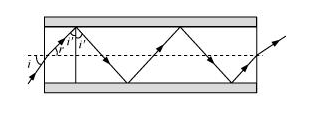Solution. (a) Refractive index of the glass fibre,$\mu_{1}=1.68$Refractive index of the outer covering of the pipe,$\mu_{2}=1.44$Angle of incidence$=i$Angle of refraction$=r$Angle of incidence at the interface$=i^{\prime}$The refractive index$(\mu)$of the inner core - outer core interface is given as:$\mu=\frac{\mu_{2}}{\mu_{1}}=\frac{1}{\sin i^{\prime}}\sin i^{\prime}=\frac{\mu_{1}}{\mu_{2}}=\frac{1.44}{1.68}=0.8571\therefore i^{\prime}=59^{\circ}$For the critical angle, total internal reflection (TIR) takes place only when$i>i^{\prime}$, i.e.,$i>59^{\circ}$Maximum angle of reflection,$r_{\max }=90^{\circ}-i^{\prime}=90^{\circ}-59^{\circ}=31^{\circ}$Let,$i_{\max }$be the maximum angle of incidence. The refractive index at the air - glass interface,$\mu_{1}=1.68$We have the relation for the maximum angles of incidence and reflection as:$\mu_{1}=\frac{\sin i_{\max }}{\sin r_{\max }}\sin i_{\max }=\mu_{1} \sin r_{\max }=1.68 \sin 31^{\circ}=1.68 \times 0.5150=0.8652\therefore i_{\max }=\sin ^{-1} 0.8652 \approx 60^{\circ}$Thus, all the rays incident at angles lying in the range$0
(b) If the outer covering of the pipe is not present, then:

Refractive index of the outer pipe, $\mu_{1}=$ Refractive index of air $=1$

For the angle of incidence $i=90^{\circ}$, we can write Snell's law at the air - pipe interface as:

$\frac{\sin i}{\sin r}=\mu_{2}=1.68$

$\sin r=\frac{\sin 90^{\circ}}{1.68}=\frac{1}{1.68}$

$r=\sin ^{-1}(0.5952)$

$=36.5^{\circ}$

$\therefore i^{\prime}=90^{\circ}-36.5^{\circ}=53.5^{\circ}$

Since $i^{\prime}>r$, all incident rays will suffer total internal reflection.

Question 18: Answer the following questions:

(a) You have learnt that plane and convex mirrors produce virtual images of objects. Can they produce real images under some circumstances? Explain.

(b) A virtual image, we always say, cannot be caught on a screen.

Yet when we ‘see’ a virtual image, we are obviously bringing it on to the ‘screen’ (i.e., the retina) of our eye. Is there a contradiction?

(c) A diver under water, looks obliquely at a fisherman standing on the bank of a lake. Would the fisherman look taller or shorter to the diver than what he actually is?

(d) Does the apparent depth of a tank of water change if viewed obliquely? If so, does the apparent depth increase or decrease?

(e) The refractive index of diamond is much greater than that of ordinary glass. Is this fact of some use to a diamond cutter?

Solution. (a) Yes

Plane and convex mirrors can produce real images as well. If the object is virtual, i.e., if the light rays converging at a point behind a plane mirror (or a convex mirror) are reflected to a point on a screen placed in front of the mirror, then a real image will be formed.

(b) No

A virtual image is formed when light rays diverge. The convex lens of the eye causes these divergent rays to converge at the retina. In this case, the virtual image serves as an object for the lens to produce a real image.

(c) The diver is in the water and the fisherman is on land (i.e., in air). Water is a denser medium than air. It is given that the diver is viewing the fisherman. This indicates that the light rays are travelling from a denser medium to a rarer medium. Hence, the refracted rays will move away from the normal. As a result, the fisherman will appear to be taller.

(d) Yes; Decrease

The apparent depth of a tank of water changes when viewed obliquely. This is because light bends on travelling from one medium to another. The apparent depth of the tank when viewed obliquely is less than the near-normal viewing.

(e) Yes

The refractive index of diamond $(2.42)$ is more than that of ordinary glass (1.5). The critical angle for diamond is less than that for glass. A diamond cutter uses a large angle of incidence to ensure that the light entering the diamond is totally reflected from its faces. This is the reason for the sparkling effect of a diamond.

Question $19$ The image of a small electric bulb fixed on the wall of a room is to be obtained on the opposite wall 3 m away by means of a large convex lens. What is the maximum possible focal length of the lens required for the purpose?

Solution. Distance between the object and the image, $d=3 \mathrm{~m}$

Maximum focal length of the convex lens $=f_{\max }$

For real images, the maximum focal length is given as:

$f_{\max }=\frac{d}{4}$

$=\frac{3}{4}=0.75 \mathrm{~m}$

Hence, for the required purpose, the maximum possible focal length of the convex lens is $0.75 \mathrm{~m}$.

Question 20: A screen is placed $90 \mathrm{~cm}$ from an object. The image of the object on the screen is formed by a convex lens at two different locations separated by $20 \mathrm{~cm}$. Determine the focal length of the lens.

Solution. Distance between the image (screen) and the object, $D=90 \mathrm{~cm}$ Distance between two locations of the convex lens, $d=20 \mathrm{~cm}$

Focal length of the lens $=f$

Focal length is related to $d$ and $D$ as:

$f=\frac{D^{2}-d^{2}}{4 D}$

$=\frac{(90)^{2}-(20)^{2}}{4 \times 90}=\frac{770}{36}=21.39 \mathrm{~cm}$

Therefore, the focal length of the convex lens is $21.39 \mathrm{~cm}$.

Question 21: (a) Determine the 'effective focal length' of the combination of the two lenses in Exercise $9.10$, if they are placed $8.0 \mathrm{~cm}$ apart with their principal axes coincident. Does the answer depend on which side of the combination a beam of parallel light is incident? Is the notion of effective focal length of this system useful at all?

(b) An object $1.5 \mathrm{~cm}$ in size is placed on the side of the convex lens in the arrangement (a) above. The distance between the object and the convex lens is $40 \mathrm{~cm}$. Determine the magnification produced by the two-lens system, and the size of the image.

Solution. Focal length of the convex lens, $f_{1}=30 \mathrm{~cm}$

Focal length of the concave lens, $f_{2}=-20 \mathrm{~cm}$

Distance between the two lenses, $d=8.0 \mathrm{~cm}$

(a) When the parallel beam of light is incident on the convex lens first:

According to the lens formula, we have:

$\frac{1}{v_{1}}-\frac{1}{u_{1}}=\frac{1}{f_{1}}$

Where,

$u_{1}=$ Object distance $=\infty$

$v_{1}=$ Image distance

$\frac{1}{v_{1}}=\frac{1}{30}-\frac{1}{\infty}=\frac{1}{30}$

$\therefore v_{1}=30 \mathrm{~cm}$

The image will act as a virtual object for the concave lens.

Applying lens formula to the concave lens, we have:’

$\frac{1}{v_{2}}-\frac{1}{u_{2}}=\frac{1}{f_{2}}$

Where,

$u_{2}=$ Object distance

$=(30-d)=30-8=22 \mathrm{~cm}$

$v_{2}=$ Image distance

$\frac{1}{v_{2}}=\frac{1}{22}-\frac{1}{20}=\frac{10-11}{220}=\frac{-1}{220}$

$\therefore v_{2}=-220 \mathrm{~cm}$

The parallel incident beam appears to diverge from a point that is $\left(220-\frac{d}{2}=220-4\right) 216 \mathrm{~cm}$ from the centre of the combination of the two lenses.

(ii) When the parallel beam of light is incident, from the left, on the concave lens first:

According to the lens formula, we have:

$\frac{1}{v_{2}}-\frac{1}{u_{2}}=\frac{1}{f_{2}}$

$\frac{1}{v_{2}}=\frac{1}{f_{2}}+\frac{1}{u_{2}}$

Where,

$u_{2}=$ Object distance $=-\infty$

$v_{2}=$ Image distance

$\frac{1}{v_{2}}=\frac{1}{-20}+\frac{1}{-\infty}=-\frac{1}{20}$

$\therefore v_{2}=-20 \mathrm{~cm}$

The image will act as a real object for the convex lens.

Applying lens formula to the convex lens, we have:

$\frac{1}{v_{1}}-\frac{1}{u_{1}}=\frac{1}{f_{1}}$

Where,

$u_{1}=$ Object distance

$=-(20+d)=-(20+8)=-28 \mathrm{~cm}$

$v_{1}=$ Image distance

$=-(20+d)=-(20+8)=-28 \mathrm{~cm}$

$v_{1}=$ Image distance

$\frac{1}{v_{1}}=\frac{1}{30}+\frac{1}{-28}=\frac{14-15}{420}=\frac{-1}{420}$

$\therefore v_{2}=-420 \mathrm{~cm}$

Hence, the parallel incident beam appear to diverge from a point that is $(420-4) 416 \mathrm{~cm}$ from the left of the centre of the combination of the two lenses.

The answer does depend on the side of the combination at which the parallel beam of light is incident. The notion of effective focal length does not seem to be useful for this combination

(b) Height of the image, $h_{1}=1.5 \mathrm{~cm}$

Object distance from the side of the convex lens, $u_{1}=-40 \mathrm{~cm}$

$\left|u_{1}\right|=40 \mathrm{~cm}$

According to the lens formula:

$\frac{1}{v_{1}}-\frac{1}{u_{1}}=\frac{1}{f_{1}}$

Where,

$v_{1}=$ Image distance

$\frac{1}{v_{1}}=\frac{1}{30}+\frac{1}{-40}=\frac{4-3}{120}=\frac{1}{120}$

$\therefore v_{1}=120 \mathrm{~cm}$

Magnification, $m=\frac{v_{1}}{\left|u_{1}\right|}$

$=\frac{120}{40}=3$

Hence, the magnification due to the convex lens is 3.

The image formed by the convex lens acts as an object for the concave lens.

According to the lens formula:

$\frac{1}{v_{2}}-\frac{1}{u_{2}}=\frac{1}{f_{2}}$

Where,

$u_{2}=$ Object distance

$=+(120-8)=112 \mathrm{~cm}$

$v_{2}=$ Image distance

$\frac{1}{v_{2}}=\frac{1}{-20}+\frac{1}{112}=\frac{-112+20}{2240}=\frac{-92}{2240}$

$\therefore v_{2}=\frac{-2240}{92} \mathrm{~cm}$

Magnification, $m^{\prime}=\left|\frac{v_{2}}{u_{2}}\right|$

$=\frac{2240}{92} \times \frac{1}{112}=\frac{20}{92}$

Hence, the magnification due to the concave lens is $\frac{20}{92}$.

The magnification produced by the combination of the two lenses is calculated as:

$m \times m^{\prime}$

$=3 \times \frac{20}{92}=\frac{60}{92}=0.652$

The magnification of the combination is given as:

$\frac{h_{2}}{h_{1}}=0.652$

$h_{2}=0.652 \times h_{1}$

Where,

$h_{1}=$ Object size $=1.5 \mathrm{~cm}$

$h_{2}=$ Size of the image

$\therefore h_{2}=0.652 \times 1.5=0.98 \mathrm{~cm}$

Hence, the height of the image is $0.98 \mathrm{~cm}$.

Question 22: At what angle should a ray of light be incident on the face of a prism of refracting angle $60^{\circ}$ so that it just suffers total internal reflection at the other face? The refractive index of the material of the prism is $1.524 .$

Solution. The incident, refracted, and emergent rays associated with a glass prism ABC are shown in the given figure.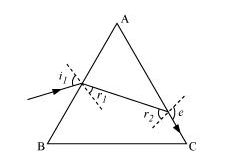Angle of prism, $\angle A=60^{\circ}$

Refractive index of the prism, $\mu=1.524$

$i_{1}=$ Incident angle

$r_{1}=$ Refracted angle

$r_{2}=$ Angle of incidence at the face $\mathrm{AC}$

$e=$ Emergent angle $=90^{\circ}$

According to Snell’s law, for face AC, we can have:

$\frac{\sin e}{\sin r_{2}}=\mu$

$\sin r_{2}=\frac{1}{\mu} \times \sin 90^{\circ}$

$=\frac{1}{1.524}=0.6562$

$\therefore r_{2}=\sin ^{-1} 0.6562 \approx 41^{\circ}$

According to Snell's law, we have the relation:

$\mu=\frac{\sin i_{1}}{\sin r_{\mathrm{i}}}$

$\sin i_{1}=\mu \sin r_{1}$

$=1.524 \times \sin 19^{\circ}=0.496$

$\therefore i_{1}=29.75^{\circ}$

Hence, the angle of incidence is $29.75^{\circ}$.

Question 23: You are given prisms made of crown glass and flint glass with a wide variety of angles. Suggest a combination of prisms which will

(a) deviate a pencil of white light without much dispersion,

(b) disperse (and displace) a pencil of white light without much deviation.

Solution. (a)Place the two prisms beside each other. Make sure that their bases are on the opposite sides of the incident white light, with their faces touching each other. When the white light is incident on the first prism, it will get dispersed. When this dispersed light is incident on the second prism, it will recombine and white light will emerge from the combination of the two prisms.

(b) Take the system of the two prisms as suggested in answer (a). Adjust (increase) the angle of the flint-glass-prism so that the deviations due to the combination of the prisms become equal. This combination will disperse the pencil of white light without much deviation

Question $24$ : For a normal eye, the far point is at infinity and the near point of distinct vision is about 25cm in front of the eye. The cornea of the eye provides a converging power of about 40 dioptres, and the least converging power of the eye-lens behind the cornea is about 20 dioptres. From this rough data estimate the range of accommodation (i.e., the range of converging power of the eye-lens) of a normal eye.

Solution. Least distance of distinct vision, $d=25 \mathrm{~cm}$

Far point of a normal eye, $d^{\prime}=\infty$

Converging power of the cornea, $P_{\mathrm{c}}=40 \mathrm{D}$

Least converging power of the eye-lens, $P_{e}=20 \mathrm{D}$

To see the objects at infinity, the eye uses its least converging power.

Power of the eye-lens, $P=P_{\mathrm{c}}+P_{\mathrm{e}}=40+20=60 \mathrm{D}$

Power of the eye-lens is given as:

$P=\frac{1}{\text { Focal length of the eye lens }(f)}$

$f=\frac{1}{P}$

$=\frac{1}{60 \mathrm{D}}$

$=\frac{100}{60}=\frac{5}{3} \mathrm{~cm}$

To focus an object at the near point, object distance $(u)=-d=-25 \mathrm{~cm}$

Focal length of the eye-lens = Distance between the cornea and the retina

$=$ Image distance

Hence, image distance, $v=\frac{5}{3} \mathrm{cn}$

According to the lens formula, we can write:

$\frac{1}{f^{\prime}}=\frac{1}{v}-\frac{1}{u}$

Where,

$f^{\prime}=$ Focal length

$\frac{1}{f^{\prime}}=\frac{3}{5}+\frac{1}{25}=\frac{15+1}{25}=\frac{16}{25} \mathrm{~cm}^{-1}$

Power, $P^{\prime}=\frac{1}{f^{\prime}} \times 100$ $=\frac{16}{25} \times 100=64 \mathrm{D}$

$\therefore$ Power of the eye-lens $=64-40=24 \mathrm{D}$

Hence, the range of accommodation of the eye-lens is from $20 \mathrm{D}$ to $24 \mathrm{D}$.

Question 25: Does short-sightedness (myopia) or long-sightedness (hypermetropia) imply necessarily that the eye has partially lost its ability of accommodation? If not, what might cause these defects of vision?

Solution. A myopic or hypermetropic person can also possess the normal ability of accommodation of the eye-lens. Myopia occurs when the eye-balls get elongated from front to back. A Hypermetropia occurs when the eye-balls get shortened. When the eye-lens loses its ability of accommodation, the defect is called presbyopia

Question 26: A myopic person has been using spectacles of power $-1.0$ dioptre for distant vision. During old age he also needs to use separate reading glass of power $+2.0$ dioptres. Explain what may have happened.

Solution. The power of the spectacles used by the myopic person, $P=-1.0 \mathrm{D}$

Focal length of the spectacles, $f=\frac{1}{P}=\frac{1}{-1 \times 10^{-2}}=-100 \mathrm{~cm}$

Hence, the far point of the person is 100 cm. He might have a normal near point of 25 cm. When he uses the spectacles, the objects placed at infinity produce virtual images at 100$\mathrm{cm}$. He uses the ability of accommodation of the eye-lens to see the objects placed between $100 \mathrm{~cm}$ and $25 \mathrm{~cm}$.

During old age, the person uses reading glasses of power, $P^{\prime}=+2 \mathrm{D}$

The ability of accommodation is lost in old age. This defect is called presbyopia. As a result, he is unable to see clearly the objects placed at $25 \mathrm{~cm}$. Question 27: A person looking at a person wearing a shirt with a pattern comprising vertical and horizontal lines is able to see the vertical lines more distinctly than the horizontal ones. What is this defect due to? How is such a defect of vision corrected?

Solution. In the given case, the person is able to see vertical lines more distinctly than horizontal lines. This means that the refracting system (cornea and eye-lens) of the eye is not working in the same way in different planes. This defect is called astigmatism. The person’s eye has enough curvature in the vertical plane. However, the curvature in the horizontal plane is insufficient. Hence, sharp images of the vertical lines are formed on the retina, but horizontal lines appear blurred. This defect can be corrected by using cylindrical lenses.

Question $28$ A man with normal near point $(25 \mathrm{~cm})$ reads a book with small print using a magnifying glass: a thin convex lens of focal length $5 \mathrm{~cm}$.

(a) What is the closest and the farthest distance at which he should keep the lens from the page so that he can read the book when viewing through the magnifying glass? (b) What is the maximum and the minimum angular magnification (magnifying power) possible using the above simple microscope?

Solution. (a) Focal length of the magnifying glass, $f=5 \mathrm{~cm}$

Least distance of distance vision, $d=25 \mathrm{~cm}$

Closest object distance $=u$

Image distance, $v=-d=-25 \mathrm{~cm}$

According to the lens formula, we have:

$\frac{1}{f}=\frac{1}{v}-\frac{1}{u}$

$\frac{1}{u}=\frac{1}{v}-\frac{1}{f}$

$=\frac{1}{-25}-\frac{1}{5}=\frac{-5-1}{25}=\frac{-6}{25}$

$\therefore u=-\frac{25}{6}=-4.167 \mathrm{~cm}$

Hence, the closest distance at which the person can read the book is $4.167 \mathrm{~cm}$.

For the object at the farthest distant $\left(u^{\prime}\right)$, the image distance $\left(v^{\prime}\right)=\infty$

According to the lens formula, we have:

$\frac{1}{f}=\frac{1}{v^{\prime}}-\frac{1}{u^{\prime}}$

$\frac{1}{u^{\prime}}=\frac{1}{\infty}-\frac{1}{5}=-\frac{1}{5}$

$\therefore u^{\prime}=-5 \mathrm{~cm}$

Hence, the farthest distance at which the person can read the book is 5 cm.

(b) Maximum angular magnification is given by the relation:

$\alpha_{\max }=\frac{d}{|u|}$

$=\frac{25}{\frac{25}{6}}=6$

Minimum angular magnification is given by the relation:

$\alpha_{\min }=\frac{d}{\left|u^{\prime}\right|}$

$=\frac{25}{5}=5$

Question 29: A card sheet divided into squares each of size 1 mm2 is being viewed at a distance of 9 cm through a magnifying glass (a converging lens of focal length 9 cm) held close to the eye.

(a) What is the magnification produced by the lens? How much is the area of each square in the virtual image?

(b) What is the angular magnification (magnifying power) of the lens?

(c) Is the magnification in (a) equal to the magnifying power in (b)?

Explain.

Solution. Note

Here we took focal Length as $10 \mathrm{~cm}$ because if we take it as $9 \mathrm{~cm}$ then image distance will be zero, which does not make any sense.

(a) Area of each square, $A=1 \mathrm{~mm}^{2}$

Object distance, $u=-9 \mathrm{~cm}$

Focal length of a converging lens, $f=9 \mathrm{~cm}$

For image distance $v$, the lens formula can be written as:

$\frac{1}{f}=\frac{1}{v}-\frac{1}{u}$

$\frac{1}{10}=\frac{1}{v}+\frac{1}{9}$

$\frac{1}{v}=-\frac{1}{90}$

$\therefore v=-90 \mathrm{~cm}$

Magnification, $m=\frac{v}{u}$

$=\frac{-90}{-9}=10$

$\therefore$ Area of each square in the virtual image $=(10)^{2} \mathrm{~A}$

$=10^{2} \times 1=100 \mathrm{~mm}^{2}$

$=1 \mathrm{~cm}^{2}$

(b) Magnifying power of the lens $=\frac{d}{|u|}=\frac{25}{9}=2.8$

(c) The magnification in (a) is not the same as the magnifying power in (b).

The magnification magnitude is $\left(\left|\frac{v}{u}\right|\right)$ and the magnifying power is $\left(\frac{d}{|u|}\right)$.

The two quantities will be equal when the image is formed at the near point $(25 \mathrm{~cm})$.

Question 30: (a) At what distance should the lens be held from the figure in Exercise 9.29 in order to view the squares distinctly with the maximum possible magnifying power?

(b) What is the magnification in this case?

(c) Is the magnification equal to the magnifying power in this case?

Explain.

Solution. (a) The maximum possible magnification is obtained when the image is formed at the near point $(d=25 \mathrm{~cm})$.

Image distance, $v=-d=-25 \mathrm{~cm}$

Focal length, $f=10 \mathrm{~cm}$

Object distance $=u$

According to the lens formula, we have:

$\frac{1}{f}=\frac{1}{v}-\frac{1}{u}$

$\frac{1}{u}=\frac{1}{v}-\frac{1}{f}$

$=\frac{1}{-25}-\frac{1}{10}=\frac{-2-5}{50}=-\frac{7}{50}$

$\therefore u=-\frac{50}{7}=-7.14 \mathrm{~cm}$

Hence, to view the squares distinctly, the lens should be kept 7.14 cm away from them.

(b) Magnification $=\left|\frac{v}{u}\right|=\frac{25}{\frac{50}{7}}=3.5$

(c) Magnifying power $=\frac{d}{u}=\frac{\frac{25}{50}}{7}=3.5$

Since the image is formed at the near point (25 cm), the magnifying power is equal to the magnitude of magnification.

Question 31: What should be the distance between the object in Exercise $9.30$ and the magnifying glass if the virtual image of each square in the figure is to have an area of $6.25 \mathrm{~mm}^{2}$. Would you be able to see the squares distinctly with your eyes very close to the magnifier?

[Note: Exercises $9.29$ to $9.31$ will help you clearly understand the difference between magnification in absolute size and the angular magnification (or magnifying power) of an instrument.]

Solution. Area of the virtual image of each square, $A=6.25 \mathrm{~mm}^{2}$

Area of each square, $A_{0}=1 \mathrm{~mm}^{2}$

Hence, the linear magnification of the object can be calculated as:

$m=\sqrt{\frac{A}{A_{0}}}$

$=\sqrt{\frac{6.25}{1}}=2.5$

But $m=\frac{\text { Image distance }(v)}{\text { Object distance }(u)}$

$\therefore v=m u$

$=2.5 u$$\ldots(1) Focal length of the magnifying glass, f=10 \mathrm{~cm} According to the lens formula, we have the relation: \frac{1}{f}=\frac{1}{v}-\frac{1}{u} \frac{1}{10}=\frac{1}{2.5 u}-\frac{1}{u}=\frac{1}{u}\left(\frac{1}{2.5}-\frac{1}{1}\right)=\frac{1}{u}\left(\frac{1-2.5}{2.5}\right) \therefore u=-\frac{1.5 \times 10}{2.5}=-6 \mathrm{~cm} And v=2.5 u =2.5 \times 6=-15 \mathrm{~cm} The virtual image is formed at a distance of 15 \mathrm{~cm}, which is less than the near point (i.e., 25 \mathrm{~cm} ) of a normal eye. Hence, it cannot be seen by the eyes distinctly. Question 32: Answer the following questions: (a) The angle subtended at the eye by an object is equal to the angle subtended at the eye by the virtual image produced by a magnifying glass. In what sense then does a magnifying glass provide angular magnification (b) In viewing through a magnifying glass, one usually positions one’s eyes very close to the lens. Does angular magnification change if the eye is moved back? (c) Magnifying power of a simple microscope is inversely proportional to the focal length of the lens. What then stops us from using a convex lens of smaller and smaller focal length and achieving greater and greater magnifying power? (d) Why must both the objective and the eyepiece of a compound microscope have short focal lengths? (e) When viewing through a compound microscope, our eyes should be positioned not on the eyepiece but a short distance away from it for best viewing. Why? How much should be that short distance between the eye and eyepiece? Solution. (a)Though the image size is bigger than the object, the angular size of the image is equal to the angular size of the object. A magnifying glass helps one see the objects placed closer than the least distance of distinct vision (i.e., 25 \mathrm{~cm} ). A closer object causes a larger angular size. A magnifying glass provides angular magnification. Without magnification, the object cannot be placed closer to the eye. With magnification, the object can be placed much closer to the eye. (b) Yes, the angular magnification changes. When the distance between the eye and a magnifying glass is increased, the angular magnification decreases a little. This is because the angle subtended at the eye is slightly less than the angle subtended at the lens. Image distance does not have any effect on angular magnification. (c) The focal length of a convex lens cannot be decreased by a greater amount. This is because making lenses having very small focal lengths is not easy. Spherical and chromatic aberrations are produced by a convex lens having a very small focal length. (d) The angular magnification produced by the eyepiece of a compound microscope is \left[\left(\frac{25}{f_{\mathrm{e}}}\right)+1\right] Where, f_{\mathrm{e}}= Focal length of the eyepiece It can be inferred that if f_{\mathrm{e}} is small, then angular magnification of the eyepiece will be large. The angular magnification of the objective lens of a compound microscope is given as \frac{1}{\left(\left|u_{o}\right| f_{0}\right)} Where, u_{\mathrm{o}}= Object distance for the objective lens f_{\mathrm{o}}= Focal length of the objective The magnification is large when u_{\mathrm{a}}>f_{\mathrm{a}}. In the case of a microscope, the object is kept close to the objective lens. Hence, the object distance is very little. Since u_{\mathrm{o}} is small, f_{\mathrm{o}} will be even smaller. Therefore, f_{\mathrm{e}} and f_{\mathrm{a}} are both small in the given condition. (e)When we place our eyes too close to the eyepiece of a compound microscope, we are unable to collect much refracted light. As a result, the field of view decreases substantially. Hence, the clarity of the image gets blurred. The best position of the eye for viewing through a compound microscope is at the eye-ring attached to the eyepiece. The precise location of the eye depends on the separation between the objective lens and the eyepiece. Question 33: An angular magnification (magnifying power) of 30 \mathrm{X} is desired using an objective of focal length 1.25 \mathrm{~cm} and an eyepiece of focal length 5 \mathrm{~cm}. How will you set up the compound microscope? Solution. Focal length of the objective lens, f_{0}=1.25 \mathrm{~cm} Focal length of the eyepiece, f_{\mathrm{e}}=5 \mathrm{~cm} Least distance of distinct vision, d=25 \mathrm{~cm} Angular magnification of the compound microscope = 30 \mathrm{X} Total magnifying power of the compound microscope, m=30 The angular magnification of the eyepiece is given by the relation: m_{\mathrm{e}}=\left(1+\frac{d}{f_{\mathrm{e}}}\right) =\left(1+\frac{25}{5}\right)=6 The angular magnification of the objective lens \left(m_{0}\right) is related to m_{e} as: m_{0} m_{\mathrm{e}}=m m_{\mathrm{o}}=\frac{m}{m_{e}} =\frac{30}{6}=5 We also have the relation: m_{0}=\frac{\text { Image distance for the objective lens }\left(v_{o}\right)}{\text { Object distance for the objective lens }\left(-u_{\mathrm{o}}\right)} 5=\frac{v_{0}}{-u_{0}} \therefore v_{\mathrm{o}}=-5 u_{\mathrm{o}}$$\ldots(1)$

Applying the lens formula for the objective lens:

$\frac{1}{f_{0}}=\frac{1}{v_{0}}-\frac{1}{u_{0}}$

$\frac{1}{1.25}=\frac{1}{-5 u_{0}}-\frac{1}{u_{0}}=\frac{-6}{5 u_{0}}$

$\therefore u_{\mathrm{o}}=\frac{-6}{5} \times 1.25=-1.5 \mathrm{~cm}$

And $v_{0}=-5 u_{0}$

$=-5 \times(-1.5)=7.5 \mathrm{~cm}$

The object should be placed 1.5 cm away from the objective lens to obtain the desired magnification.

Applying the lens formula for the eyepiece:

$\frac{1}{v_{\mathrm{c}}}-\frac{1}{u_{\mathrm{e}}}=\frac{1}{f_{\mathrm{c}}}$

Where,

$v_{\mathrm{e}}=$ Image distance for the eyepiece $=-d=-25 \mathrm{~cm}$

$u_{\mathrm{e}}=$ Object distance for the eyepiece

$\frac{1}{u_{e}}=\frac{1}{v_{e}}-\frac{1}{f_{e}}$

$=\frac{-1}{25}-\frac{1}{5}=-\frac{6}{25}$

$\therefore u_{\mathrm{e}}=-4.17 \mathrm{~cm}$

Separation between the objective lens and the eyepiece $=\left|u_{\mathrm{e}}\right|+\left|v_{\mathrm{o}}\right|$

$=4.17+7.5$

$=11.67 \mathrm{~cm}$

Therefore, the separation between the objective lens and the eyepiece should be 11.67 cm.

Question 34: A small telescope has an objective lens of focal length 140 cm and an eyepiece of focal length 5.0 cm. What is the magnifying power of the telescope for viewing distant objects when

(a) the telescope is in normal adjustment (i.e., when the final image is at infinity)?

(b) the final image is formed at the least distance of distinct vision $(25 \mathrm{~cm}) ?$

Solution. Focal length of the objective lens, $f_{\mathrm{o}}=140 \mathrm{~cm}$

Focal length of the eyepiece, $f_{\mathrm{e}}=5 \mathrm{~cm}$

Least distance of distinct vision, $d=25 \mathrm{~cm}$

(a) When the telescope is in normal adjustment, its magnityıng power is given as:

$m=\frac{f_{0}}{f_{e}}$

$=\frac{140}{5}=28$ (b) When the final image is formed at d,the magnifying power of the telescope is given as:

$\frac{f_{\mathrm{o}}}{f_{\mathrm{e}}}\left[1+\frac{f_{\mathrm{c}}}{d}\right]$

$=\frac{140}{5}\left[1+\frac{5}{25}\right]$

$=28[1+0.2]$

$=28 \times 1.2=33.6$

Question 35: (a) For the telescope described in Exercise $9.34$ (a), what is the separation between the objective lens and the eyepiece?

(b) If this telescope is used to view a 100 m tall tower 3 km away, what is the height of the image of the tower formed by the objective lens?

(c) What is the height of the final image of the tower if it is formed at 25 cm?

Solution. Focal length of the objective lens, $f_{0}=140 \mathrm{~cm}$

Focal length of the eyepiece, $f_{\mathrm{e}}=5 \mathrm{~cm}$

(a) In normal adjustment, the separation between the objective lens and the eyepiece $=f_{\mathrm{o}}+f_{\mathrm{e}}=140+5=145 \mathrm{~cm}$

(b) Height of the tower, $h_{1}=100 \mathrm{~m}$

Distance of the tower (object) from the telescope, $u=3 \mathrm{~km}=3000 \mathrm{~m}$

The angle subtended by the tower at the telescope is given as:

$\theta=\frac{h_{1}}{u}$

$=\frac{100}{3000}=\frac{1}{30} \mathrm{rad}$

The angle subtended by the image produced by the objective lens is given as:

$\theta=\frac{h_{2}}{f_{0}}=\frac{h_{2}}{140} \mathrm{rad}$

Where,

$h_{2}=$ Height of the image of the tower formed by the objective lens

$\frac{1}{30}=\frac{h_{2}}{140}$

$\therefore h_{2}=\frac{140}{30}=4.7 \mathrm{~cm}$

Therefore, the objective lens forms a 4.7 cm tall image of the tower.

(c) Image is formed at a distance, d = 25 cm

The magnification of the eyepiece is given by the relation:

$m=1+\frac{d}{f_{\mathrm{e}}}$

$=1+\frac{25}{5}=1+5=6$

Height of the final image $=m h_{2}=6 \times 4.7=28.2 \mathrm{~cm}$

Hence, the height of the final image of the tower is $28.2 \mathrm{~cm}$.

Question $36$ : A Cassegrain telescope uses two mirrors as shown in Fig. 9.33. Such a telescope is built with the mirrors $20 \mathrm{~mm}$ apart. If the radius of curvature of the large mirror is 220 mm and the small mirror is $140 \mathrm{~mm}$, where will the final image of an object at infinity be?

Solution. The following figure shows a Cassegrain telescope consisting of a concave mirror and a convex mirror.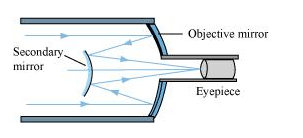Distance between the objective mirror and the secondary mirror, $d=20 \mathrm{~mm}$

Radius of curvature of the objective mirror, $R_{1}=220 \mathrm{~mm}$

Hence, focal length of the objective mirror, $f_{1}=\frac{R_{1}}{2}=110 \mathrm{~mm}$

Radius of curvature of the secondary mirror, $R_{1}=140 \mathrm{~mm}$

Hence, focal length of the secondary mirror, $f_{2}=\frac{R_{2}}{2}=\frac{140}{2}=70 \mathrm{~mm}$

The image of an object placed at infinity, formed by the objective mirror, will act as a virtual object for the secondary mirror.

Hence, the virtual object distance for the secondary mirror, $u=f_{1}-d$

$=110-20$

$=90 \mathrm{~mm}$

Applying the mirror formula for the secondary mirror, we can calculate image distance (v)as:

$\frac{1}{v}+\frac{1}{u}=\frac{1}{f_{2}}$

$\frac{1}{v}=\frac{1}{f_{2}}-\frac{1}{u}$

$=\frac{1}{70}-\frac{1}{90}=\frac{9-7}{630}=\frac{2}{630}$

$\therefore v=\frac{630}{2}=315 \mathrm{~mm}$

Hence, the final image will be formed 315 mm away from the secondary mirror.

Question 37: Light incident normally on a plane mirror attached to a galvanometer

coil retraces backwards as shown in Fig. $9.36 .$ A current in the coil produces a deflection of $3.5^{\circ}$ of the mirror. What is the displacement of the reflected spot of light on a screen placed $1.5 \mathrm{~m}$ away?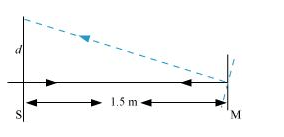Solution. Angle of deflection, $\theta=3.5^{\circ}$

Distance of the screen from the mirror, $D=1.5 \mathrm{~m}$

The reflected rays get deflected by an amount twice the angle of deflection i.e., $2 \theta=7.0^{\circ}$

The displacement $(d)$ of the reflected spot of light on the screen is given as:

$\tan 2 \theta=\frac{d}{1.5}$

$\therefore d=1.5 \times \tan 7^{\circ}=0.184 \mathrm{~m}=18.4 \mathrm{~cm}$

Hence, the displacement of the reflected spot of light is $18.4 \mathrm{~cm}$.

Question 38: Figure $9.37$ shows an equiconvex lens (of refractive index $1.50)$ in contact with a liquid layer on top of a plane mirror. A small needle with its tip on the principal axis is moved along the axis until its inverted image is found at the position of the needle. The distance of the needle from the lens is measured to be $45.0 \mathrm{~cm}$. The liquid is removed and the experiment is repeated. The new distance is measured to be $30.0 \mathrm{~cm}$. What is the refractive index of the liquid?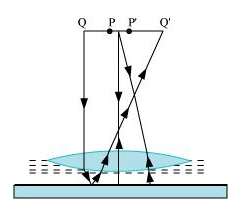Solution. Focal length of the convex lens, $f_{1}=30 \mathrm{~cm}$

The liquid acts as a mirror. Focal length of the liquid $=f_{2}$c

Focal length of the system (convex lens + liquid), $f=45 \mathrm{~cm}$

For a pair of optical systems placed in contact, the equivalent focal length is given as:

$\frac{1}{f}=\frac{1}{f_{1}}+\frac{1}{f_{2}}$;

$\frac{1}{f_{2}}=\frac{1}{f}-\frac{1}{f_{1}}$

$=\frac{1}{45}-\frac{1}{30}=-\frac{1}{90}$

$\therefore f_{2}=-90 \mathrm{~cm}$

Let the refractive index of the lens be $\mu_{1}$ and the radius of curvature of one surface be R. Hence, the radius of curvature of the other surface is −R. R can be obtained using the relation:

R can be obtained using the relation:

$\frac{1}{f_{1}}=\left(\mu_{1}-1\right)\left(\frac{1}{R}+\frac{1}{-R}\right)$

$\frac{1}{30}=(1.5-1)\left(\frac{2}{R}\right)$

$\therefore R=\frac{30}{0.5 \times 2}=30 \mathrm{~cm}$

Let $\mu_{2}$ be the refractive index of the liquid.

Radius of curvature of the liquid on the side of the plane mirror $=\infty$

Radius of curvature of the liquid on the side of the lens, $R=-30 \mathrm{~cm}$

The value of $\mu_{2}$ can be calculated using the relation:

$\frac{1}{f_{2}}=\left(\mu_{2}-1\right)\left[\frac{1}{-R}-\frac{1}{\infty}\right]$

$\frac{-1}{90}=\left(\mu_{2}-1\right)\left[\frac{1}{+30}-0\right]$

$\mu_{2}-1=\frac{1}{3}$

$\therefore \mu_{2}=\frac{4}{3}=1.33$

Hence, the refractive index of the liquid is $1.33 .$